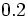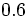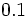# Limiting coefficient of static friction

## Definition

Given two surfaces, the limiting coefficient of static friction between them, denoted$\mu_s$ or$\mu_l$, is defined as the number such that the static friction between any two bodies with these two surfaces respectively as the surfaces of contact cannot exceed$\mu_sN$, where$N$ is the normal force between the two bodies.

The notion of limiting coefficient of static friction arises as part of the Coulomb model of friction.

## Units and dimensions

The limiting coefficient of static friction is a dimensionless scalar and does not need units.

## Constitutive factors and measurement

The limiting coefficient of static friction between two surfaces depends on the two surfaces, and as such, should be the same for the same pair of surfaces. However, the coefficient depends on a number of factors such as dampness, scratches, or minor surface changes, hence the measurement for the same pair of surfaces may differ with time.

For this reason, the limiting coefficient of static friction for a pair of surfaces must be determined empirically, through measurement. However, a reasonable approximate value can be estimated based on the nature of the surfaces.

## Range of values

The limiting coefficient of static friction typically takes values in the range of$0.2$ to$0.6$. In most cases, the limiting coefficient of static friction is less than$1$, but there could be situations where it is greater than$1$. For very slippery surfaces, the limiting coefficient of static friction may be less than$0.1$.